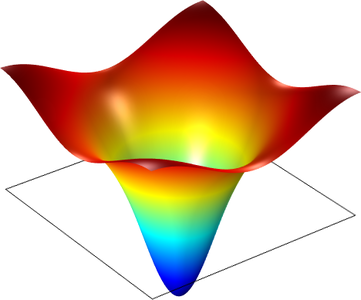# Application Gallery

## Band-Gap Analysis of a Photonic Crystal

Application ID: 798

This model investigates the wave propagation in a photonic crystal that consists of GaAs pillars placed equidistant from each other.

The distance between the pillars determines a relationship between the wave number and the frequency of the light, which prevents light of certain wavelengths propagating inside the crystal structure. This frequency range is called the photonic bandgap. There are several bandgaps for a certain structure, and this model extracts the bandgaps for the lowest bands of the crystal.

There are two main complications with a bandgap analysis. Firstly, the refractive index of GaAs is frequency dependent. Secondly, the wave vector must be ramped for the band diagram.

Although you can solve for each of these complications with the eigenvalue solver separately, the two combined make it difficult without a script. However, it is possible to solve a nonlinear problem with the stationary solver, using the eigenvalue as an unknown. The equation for the eigenvalue is therefore a normalization of the electric field, so the average field is unity over the domain. The nonlinear solver finds the correct eigenvalue with an updated refractive index to the found eigenvalue. Furthermore, the parametric solver can sweep the wave vector, k.

The model performs an eigenvalue analysis, using the nonlinear solver with an extra normalization equation for the eigenvalue.This model example illustrates applications of this type that would nominally be built using the following products: